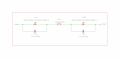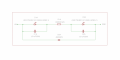# Advice on High Intensity LED Current, Reverse Polarity Protection & Temperature

#### SnowCrash

Joined Apr 6, 2016
31
Hi,

Background
My basic circuit consists of 2 x 'mid-power' high-intensity warm white LEDs hooked-up in series with a current limiting resistor sitting in-between and 2 x ceramic bypass capacitors (one for each LED), all of which are driven by a 12VDC power supply, as depicted in the following drawing:Despite the simplicity of this circuit, I'm unclear about several points and would very much appreciate the input of more experienced members of this forum.

Circuit Technical Details:
D1A
& D2A : high-intensity warm white smd LEDs (GW PSLMS1.EC-GSGU-5O8Q-1, product page, datasheet)
R1A : smd resistor (100R/0.5W, 0805 package)
C1A & C2A : smd ceramic capacitor (0.1uF/50V, 0805 package)

Reverse Polarity Protection
I'd like to add reverse current protection for the LEDs in this circuit. However, due to severe space limitations, the best I can do atm is fit a single diode in reverse-series with the power lines as shown in the follow diagram:The diode which I have on hand and would like to use is the following:

D3A : smd general purpose diode (BAS321, 0805 package, product page, datasheet)

Space limitations also mean I cannot fit any additional component into the circuit.

Questions
Given all of the above, these are the open questions I'm hoping to get advice on:

(1) Would the said diode (D3A), fitted in the above position, provide decent reverse current protection for the LEDs? and if not, what would be the most minimal adjustment I could make to achieve such protection?

(2) The 100R/0.5W resistor (R1A) was selected so as to drive the 2 LEDs at 0.65mA (if I'm not mistaken, at this current level, each LED introduces 3.3V forward voltage drop, so there should be plenty of headroom with a 12V power supply). Is this calculation correct? and, would this current level be a good choice for long-term operation these LEDs? and, finally, if not, what would be a better current level and why?

(3) The 2 LEDs are packed into a very compact space and I'm worried about their temperature rising to a level that would compromise the encasing structure (made of 3D printed PLA or similar material). Can someone help me figure out from the datasheet what would be the temperature of the LEDs when operating in the above conditions (0.65mA)? Moreover, any additional advice on thermal considerations re this circuit would be much appreciated.

(4) Of course, any input/correction/advice on the circuit as a whole would be very welcome!

Thank you very much in advance,
SnowCrash

#### Dodgydave

Joined Jun 22, 2012
9,868
The Bas321 diode is only 250mA rated, i would use a 1N5401 series diode.

#### SnowCrash

Joined Apr 6, 2016
31
The Bas321 diode is only 250mA rated, i would use a 1N5401 series diode.
Thanks for the feedback, Dodgydave!

Would this diode be more suitable?

PMEG2020EJ - Schottky Diode 20V/2A (product page, datasheet)

#### Dodgydave

Joined Jun 22, 2012
9,868
Yes.

•SnowCrash

#### SnowCrash

Joined Apr 6, 2016
31
Cool, thanks Dodgydave!

Could anyone help me with my other questions please?

#### SnowCrash

Joined Apr 6, 2016
31
Your leds are taking 3.3V @ 65mA, that's 2.2W, your series resistor is going to be rated at 5W Minimum ideally...

Total wattage 12V @65mA is 8W approx gives 6W for resistor droppage.
Hi Dodgydave,

My calculations for the LEDs are as follows:

Single LED Power Dissipation:
PLED Forward Voltage x LED Forward Current = Watts)
Pled = Vf x I = 3.3 x 0.065 = 214.5mW

Total LEDs Power Dissipation:
Ptotal = Vf x n x I = 3.3 x 2 x 0.065 = 429mW

My calculations for the resistor are as follows:

Resistor Value:
Going by the formula for calculating the resistor value for LEDs in series:
R = (Vs - (Vf x n)) / I = (12 - (3.3 x 2)) / 0.065 = 83.1R
Then rounding this upward to the nearest common resistor value: 83.1R -> 100R

Resistor Power Dissipation:
using the formula for LED Total Forward Current Squared divided by the Resistor Value)
(0.065^2) * 100 = 422.5mW

These values are very different from what you're suggesting... so where (& why) do we diverge so radically? are my calculations totally wrong?

#### Dodgydave

Joined Jun 22, 2012
9,868
Hi Dodgydave,

My calculations for the LEDs are as follows:

Single LED Power Dissipation:
PLED Forward Voltage x LED Forward Current = Watts)
Pled = Vf x I = 3.3 x 0.065 = 214.5mW

Total LEDs Power Dissipation:
Ptotal = Vf x n x I = 3.3 x 2 x 0.065 = 429mW

My calculations for the resistor are as follows:

Resistor Value:
Going by the formula for calculating the resistor value for LEDs in series:
R = (Vs - (Vf x n)) / I = (12 - (3.3 x 2)) / 0.065 = 83.1R
Then rounding this upward to the nearest common resistor value: 83.1R -> 100R

Resistor Power Dissipation:
using the formula for LED Total Forward Current Squared divided by the Resistor Value)
(0.065^2) * 100 = 422.5mW

These values are very different from what you're suggesting... so where (& why) do we diverge so radically? are my calculations totally wrong?
Sorry my mistake, 12V @65mA is 780mW total, leds use 214mW, that's 566 mW for resistor...

#### SnowCrash

Joined Apr 6, 2016
31
Sorry my mistake, 12V @65mA is 780mW total, leds use 214mW, that's 566 mW for resistor...
No worries, happy to know the calculations are ok#### ian field

Joined Oct 27, 2012
6,539
Thanks for the feedback, Dodgydave!

Would this diode be more suitable?

PMEG2020EJ - Schottky Diode 20V/2A (product page, datasheet)
SB diodes have significant leakage. It may not be a concern, but I'd use a cheaper basic silicon for series protection.

Inverse parallel protection is far more popular - an SB diode would clamp reverse voltage at a lower value, but basic silicon is perfectly adequate.

#### SnowCrash

Joined Apr 6, 2016
31
Thanks for the input, ian field!

SB diodes have significant leakage. It may not be a concern, but I'd use a cheaper basic silicon for series protection.
Just to clarify, by 'SB diode' do you mean Schottky diode?

Inverse parallel protection is far more popular - an SB diode would clamp reverse voltage at a lower value, but basic silicon is perfectly adequate.
Not sure I understand the first part: doesn't the setup I suggested above constitute 'inverse parallel protection'? and, if not, could you please explain in more detail how can that kind of protection be achieved?

Also, could you please give me an example of the kind of silicon diode you have in mind (preferably in a SOD323 / 0805 package or similar) that would be suitable here?

#### SnowCrash

Joined Apr 6, 2016
31
SB diodes have significant leakage. It may not be a concern, but I'd use a cheaper basic silicon for series protection.

Inverse parallel protection is far more popular - an SB diode would clamp reverse voltage at a lower value, but basic silicon is perfectly adequate.
Hi Ian,
Any chance you could find a moment to respond to my request for clarifications to your post above?
Thanks,
SnowCrash

#### ian field

Joined Oct 27, 2012
6,539
Hi Ian,
Any chance you could find a moment to respond to my request for clarifications to your post above?
Thanks,
SnowCrash
Maybe you could clarify what you're trying to accomplish.